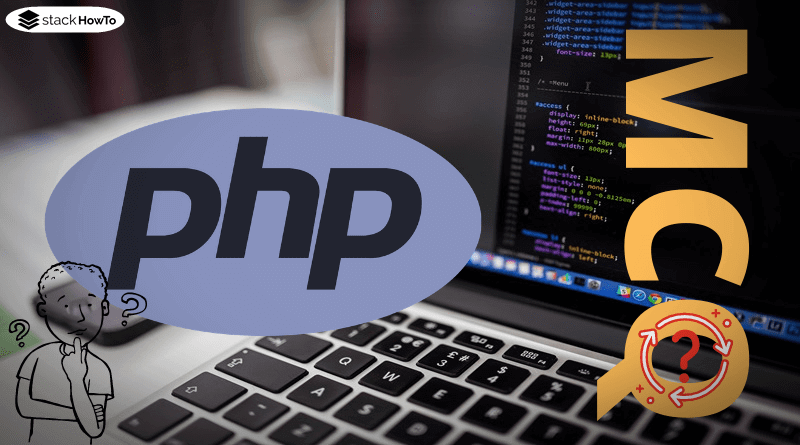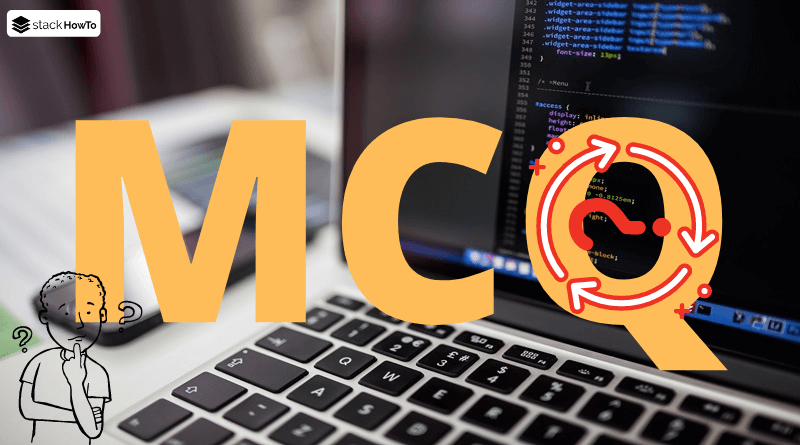php

# PHP – Static Variables

In this tutorial, we’re going to see how to use the static variables in functions in PHP.

If the scope of a function is abandoned, then all variables defined within this function are usually lost. The next time the function is called, all local variables must therefore be defined again. Sometimes, however, this is not desired, for example, if computationally intensive tasks are only to be carried out once and then temporarily stored. In order to ensure that certain local variables retain their values over several calls to a function, these can be marked as “static”. After the end of the function, these are not available outside the scope of the function, but the next time they are called they can be accessed again within the function – as if the function had not been terminated, but simply started again at the beginning.

###### Example with a static variable:

In the example below, the static variable \$x “survives” the end of the function.

```<?php
function echoStaticVar() {
// define static variable \$x
// Important: This is only executed once
// set to 0 every time a function is called
static \$x = 0;

echo("\$x\n"); // output
\$x++; // increase by 1
}

echoStaticVar();
echoStaticVar();
echoStaticVar();
?>```

Output:

```0
1
2```PHP MCQ – Multiple Choice Questions and Answers – Basics – Part 1This collection of PHP Multiple Choice Questions and Answers (MCQs): Quizzes & Practice Tests with Answer focuses on “PHP basic”.   1. In PHP, variables…Read More

###### Example : No access from “outside”

The static variables of a function cannot be accessed from outside the scope. They are only available within the function.

```<?php
function echoStaticVar() {
static \$x = 0;
\$x = 50;
echo("\$x\n");
}

echoStaticVar();
// generates an error because \$x is only available in echoStaticVar()
var_dump(\$x);
?>```

Output:

```50
Notice:  Undefined variable: x in [...][...] on line 10
NULL```

###### Example : Static keyword must be used every time it is called up

The “static” keyword can in principle be used anywhere in the function, but must always be placed before the first access to the variable. Otherwise the variable may contain incorrect values or be completely missing. In the next example, the “static” keyword is only used when the function is called for the first time, after that it is not used anymore. The result is that PHP complains about \$x not being defined starting with the second call.

```<?php
\$GLOBALS['called'] = false;

function echoStaticVar() {
if (!\$GLOBALS['called']) {
static \$x = 0;
\$GLOBALS['called'] = true;
}

echo("\$x\n");
\$x++;
}

echoStaticVar();
echoStaticVar();
?>```

Output:

```0
Notice:  Undefined variable: x in [...][...] on line 10
Notice:  Undefined variable: x in [...][...] on line 11```

This now raises the question what happens if you mark a variable as static for some function calls and use it as a “normal” local variable for some calls. As the following example shows, the static variable is not lost.

```<?php
\$GLOBALS['calls'] = 0;

function echoStaticVar() {
// Mark \$x as static with every second call
if (\$GLOBALS['calls'] % 2 === 0) {
static \$x = 0;
} else {
\$x = 0;
}
\$GLOBALS['calls']++;

echo("\$x\n");
\$x++;
}

echoStaticVar();
echoStaticVar();
echoStaticVar();
echoStaticVar();
echoStaticVar();
echoStaticVar();
?>```

Output:

```0
0
1
0
2
0```

You might ask yourself, what kind of behaviour it leads to, if you first define a local variable within a function call and then mark it as static. The next example tries this and it turns out that the variable changes back to its static value when using the “static” keyword:

```<?php
\$GLOBALS['calls'] = 0;

function echoStaticVar() {
// first define local variable with name \$x
\$x = 0;
echo("\$x\n");

// then define static variables with the same name
static \$x = 0;
echo("\$x\n");
\$x++;
}

echoStaticVar();
echoStaticVar();
echoStaticVar();
echoStaticVar();
?>```

Output:

```0
0
0
1
0
2
0
3```PHP global variable with ExampleVariables usually have a scope, so variables defined in one function are not available for other functions. However, it is also possible to place variables…Read MoreMCQPractice competitive and technical Multiple Choice Questions and Answers (MCQs) with simple and logical explanations to prepare for tests and interviews.Read More